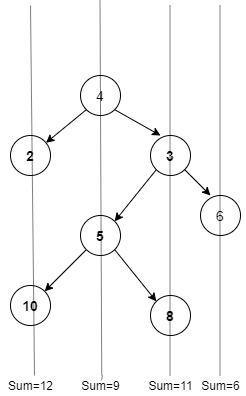# Vertical Sum in BST

Posted: 27 Jul, 2021
Difficulty: Moderate

## PROBLEM STATEMENT

#### You are required to print the sum along vertical lines from the leftmost node and moving to the rightmost node.

##### For Example :
``````Consider the following Binary Tree:
````````````So the final answer is
12 9 11 6
``````
##### Input Format :
``````The first line of input contains an integer ‘T’ denoting the number of test cases.
The next ‘T’ lines represent the ‘T’ test cases.

The first line of each test case contains the elements of the tree in the level order form separated by a single space. If any node does not have a left or right child, take -1 in its place.
For example, the input for the tree depicted in the below image would be :
````````````1
2 3
4 -1 5 6
-1 7 -1 -1 -1 -1
-1 -1
``````

#### Explanation :

``````Level 1 :
The root node of the tree is 1

Level 2 :
Left child of 1 = 2
Right child of 1 = 3

Level 3 :
Left child of 2 = 4
Right child of 2 = null (-1)
Left child of 3 = 5
Right child of 3 = 6

Level 4 :
Left child of 4 = null (-1)
Right child of 4 = 7
Left child of 5 = null (-1)
Right child of 5 = null (-1)
Left child of 6 = null (-1)
Right child of 6 = null (-1)

Level 5 :
Left child of 7 = null (-1)
Right child of 7 = null (-1)

The first not-null node (of the previous level) is treated as the parent of the first two nodes of the current level. The second not-null node (of the previous level) is treated as the parent node for the next two nodes of the current level and so on.
The input ends when all nodes at the last level are null (-1).
``````
##### Note :
``````The above format was just to provide clarity on how the input is formed for a given tree.
The sequence will be put together in a single line separated by a single space. Hence, for the above-depicted tree, the input will be given as:

1 2 3 4 -1 5 6 -1 7 -1 -1 -1 -1 -1 -1
``````
##### Output Format :
``````For each test case, print the vertical sum of node values separated by a space in a separate line.
``````
##### Constraints :
``````1 <= T <= 10
1 <= N <= 3000
1 <= node data <= 10^9

Time Limit: 1sec
``````
##### Note :
``````You do not need to print anything, it has already been taken care of. Just implement the given function.
``````Approach 1

First, we see below example before discussing the approach:

For the above given binary tree:

1 is the root node, we say its horizontal distance = 0.

Since 2 lies to the left of 1, its horizontal distance from 1 is 0-1= -1

3 lies to the right of 1, its horizontal distance = 0+1 = 1

Similarly, horizontal distance of 4 = Horizontal distance of 2 - 1= -1-1=-2

Horizontal distance of 5 = Horizontal distance of 2 + 1=  -1+1 = 0

Horizontal distance of 6 = Horizontal distance of 3 - 1 i.e 1-1 =0

Horizontal distance of 7 = Horizontal distance of 3 +1 i.e 1+1 = 2

So Nodes at horizontal distance -2 = 4 , Sum=4,

Nodes at horizontal distance -1 = 2 , Sum=2,

Nodes at horizontal distance  0 = 1,5,6 ,Sum=12 (1+5+6),

Nodes at horizontal distance 1= 3 , Sum=3,

And Nodes at horizontal distance 2= 7, Sum=7

So the final answer is  4 2 12 3 7

Algorithm : -

• So we use a map having {horizontalDistance,sumOfNodes} as the key-value pair.
• Now on traversing the tree, we check whether the current calculated horizontal distance is present on the map.
• If it is present on the map, we increment that key’s value by the node value.
• Otherwise, we make a new key, value pair in the map having the current horizontal distance as the key and the node value as its value.
• Then we move on to traverse the remaining subtree. We have to pass the horizontal distance as an argument while traversing the tree.
• Note that the horizontal distance gets increased by 1 when we move to a right child whereas it gets decreased by 1 when we move to a left child.
• When the tree is completely traversed, we traverse the map and print the map values in the order of least horizontal distance value to the greatest horizontal distance value.

We will use an ordered map as we need to print the sequence in the sorted order of horizontal distance.